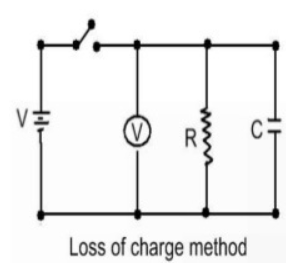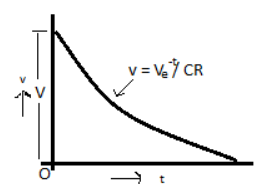### Loss of charge Method

In Loss of charge method' the insulation resistance R to be measured is connected in parallel with a capacitor C and an electrostatic voltmeter. The capacitor is charged to some suitable voltage, by means of a battery having voltage V and is then allowed to discharge through the resistance. The terminal voltage is observed over a considerable period of time during discharge.The voltage across the capacitor at any instant t after the application of voltage is

V = V exp(-t/ CR)

or  V/ v = exp(-t/ CR)

or Insulation resistance

R  =  t / {C log V/v}

=  0.4343t /{C log V/v}

The variation of voltage v with time shown in below figure:From the equation above, it follows that if V,v, C and t are known the value of R can be computed. If the resistance R is very large the time for an appreciable fall in voltage is very large and thus this process may become time-consuming. Also the voltage-time curve will thus be very flat and unless great care is taken in measuring voltage at the beginning and end of the time 'r', a serious error may be made in the ratio V / v causing a considerable corresponding error in the measured value of R. More accurate results may be obtained by change in the voltage V-v directly and calling this change as e, the expression for R becomes

The test is then repeated with the unknown resistance R, disconnected and the capacitor discharging through R1. The value of R1 obtained from this second test and substituted into the expression

in order to get the value of R.

The leakage resistance of the voltmeter, unless very high, should also be taken into consideration.